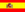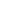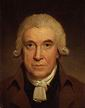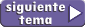home | más de trabajo y energía | otros temas de Física | lecciones del maestro Ciruela | tonterías | @NO ME SALEN
APUNTES TEORICOS DE FÍSICA DEL CBC
(Conservation laws)POWER

If you go upstairs four floors slowly, step by step, with several breaks in between... taking about 20 minutes... it's clear that you were not in a hurry at all. But if you go up running all along, two stairs by step, or three stairs by step, with your tongue out of your mouth and in record time... things become very different, and I would like to see if you're able to. Indeed, this is about two very different physical processes. However, the variation of energy in both cases are the same. The essential difference goes through the speed with which the energy variation ocurred.

In a wide range of physical transformations it doesn't matter how much work is done, or how much the energy of a system changes.

The magnitude that describes how quick is an energy shift, is the power, which is defined this way: the ratio between a variation of energy and the time range in which the variation occurs.

 Pot = ΔE / Δt average power

The units that works to measure the power can be any unit of power obtained by the ratio of energy and any unit that measures time. In the International System:

[Pot] = J / s = W        (watt)

because it is a very important magnitude, the unit which measures has an own name, adopted by a scottish engineer, James Watt (1736-1819) in his honor, known as the creator of the steam engine ... which we're going to talk again.

It can also be defined as the ratio between work done and the time range in which it was performed:James Watt
 Pot = L / Δt average power

Both definitions have the issue of that if we want to know (or estimate) the power, it's necessary to know the total work done and the total time taken, in order to calculate the ratio.

Although, recalling that the work is the product between force and displacement...

Pot = F . Δx / Δt

 Pot = F . v instant power

This last definition of power allows us to do an instant calculation, since it doesn't depend on any interval of any kind.

At any instant in which we know the value of a force that is working, and the speed with which the displacement occurs for that work, we can find the strength, which (although it is about the same magnitude) is called instant power.

If you go up all four floors of the staircase in this way: the first floor you go like the road-runner, and the following three in turtle steps, it is clear that the power with which you went up the first floor is very different from the following three. The calculation of instant power can be much more descriptive in this upward movement than the calculation taking the total variations.  In case of any process may occur at variable power, the firsts definitions would be named average power.

IMPORTANT GOSSIP:

• Power plants supply electricity to the population, they measure their performance in power terms, since what it matters in these cases is not the amount of energy delivered, but in how long is delivered the amount of energy to be delivered. The set of power generation plants in Argentina occurs at a rate of 25,000 MW (installed capacity).
• A space shuttle needs to consume energy at a rate of 33,000 MW during liftoff.

TRYCKY QUESTIONS:

• How much power can a horse develop about?
• What other power units exist?
• What power does the human heart consume? What power does it produce?
• How much power gives the Sun to Earth? How much power to Argentina? How much power per square meter?
• Using all the energy resources of Argentina, how many 25 watt light bulbs could be lit at the same time?Translated by Matías Llofriú. Algunos derechos reservados. Se permite su reproducción citando la fuente. Última actualización jun-09. Buenos Aires, Argentina.